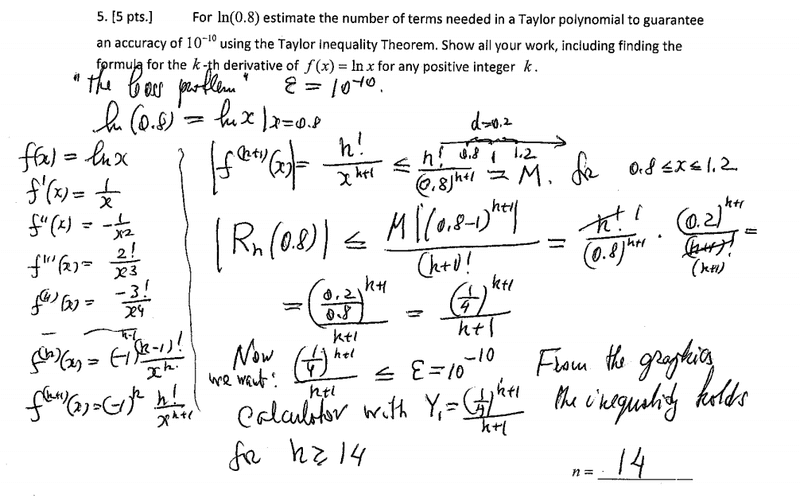# Estimate number of terms needed for taylor polynomial

timnswede

## Homework Statement

For ln(.8) estimate the number of terms needed in a Taylor polynomial to guarantee an accuracy of 10^-10 using the Taylor inequality theorem.

## Homework Equations

|Rn(x)|<[M(|x-a|)^n+1]/(n+1)! for |x-a|<d.

## The Attempt at a Solution

All I've done so far is take a couple derivatives of ln(x):
1/x
-1/x^2
2!/x^3
-3!/x^4
and made a general (-1)^(n+1) (n-1)!/(x^n)
At this point I am lost, am I supposed to assume a=0? Then M, which is the absolute value of the n+1 derivative would be n!/.8^(n+1). And plugging that into the Taylor inequality equation the .8^(n+1) would cancel and so would the n! and I would be left with 1/n+1<10^-10, which gives me a really big number, even though the answer should be 14. What am I missing?

EDIT: It looks like there might be some missing information. My professor sent the solution out, and to me it looks like he is using a=1. Can someone confirm that?Last edited: